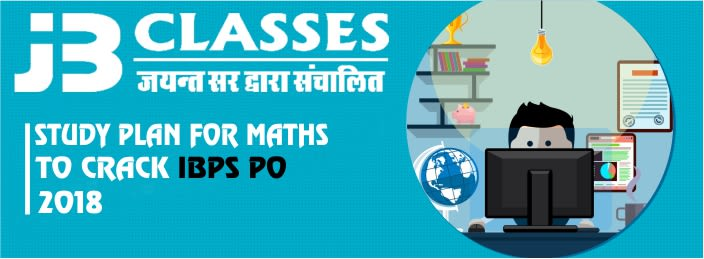IBPS PO 2018 is scheduled to take place from 18th September to 22nd September of this year. IBPS PO is one of the most awaited banking exams for the aspirants in the banking sector. Changes in the difficulty level of Quant have been reflected in exams such as SBI PO 2018, BOB PO 2018 and more. In all these exams, the pattern of Quant has remained the same but the difficulty level of questions has increased. Therefore, it becomes necessary for you to not just study more but also study smartly. In this article, we will provide you with how to prepare tips for IBPS PO Quant.
Quantitative Aptitude involves a lot of calculations. This can become frustrating sometimes. However, this occasional dullness of Quant can be removed by studying it with quizzes and reading. Make sure that your practice is a blend of reading important tips for the section as well as practising sums. The best way to prepare is following a strategy prioritising topic that are easy and have high weightage.
Also,

• Remember that there is negative marking. Every wrong answer will deduct 0.25 of the marks assigned to the question from your paper.
• Give each section equal preparation time since sectional timing is introduced this year.
Go through the exam pattern before you begin this to ensure complete familiarity what you are going to face in exams.

While preparing, as will be understood by the tips below, there are too many topics in IBPS PO Quant section. Therefore, what you have to do is practice the questions in a particular order. This order will be in such a way:
1. More Weightage + Easy Questions
2. Time Consuming + Easy Questions
3. More Weightage + Difficult Questions
4. Time Consuming + Difficult Questions
1.
 Topic Weightage Simplification 5 Average 1 Percentage 1 – 2 Ratio & Proportion 1 – 2 Data Interpretation 5 – 10 Mensuration 1 – 3 Quadratic Equations 0 – 5 Interest 1 – 2 Problems Related to Age 0 – 1 Profit & Loss 1 – 3 Number Series 5 Speed, Time, Distance 1 – 3 Time & Work 1 – 2 Number System 0 – 1 Data Sufficiency 0 – 5 Linear Equations 0 – 1 Permutations & Combination/ Probability 0 – 1 Mixtures & Alligitions 1

Before you are go through the preparation strategies we give here, make sure you are completely familiar with the syllabus. This will help you to know what exactly to expect.

## IBPS PO – Prepare Easy Topics

1. Topics: Simplification, Average, Percentage, Ratio & Proportion, Interest, Profit & Loss.
2. These topics are very scoring in nature.
3. In terms of solving, they are less time consuming.
4. Therefore, more accuracy is possible in the questions devoted to these topics.

#### Strategies:

• Simplification –
• Start targeting for these questions early in the preparations.
• Concepts are easy to understand and so you should keep yourself familiar with them.
• Practice quick mental calculations to save time while solving.
• Try using short tricks and techniques for faster calculations.
• Practice a mix of problems on BODMAS Rule, Approximations, Square & Cubes, Surds & Indices, Decimals and Percentages.
• Average, Percentage, Ratio & Proportion, Interest, Profit & Loss –
• Begin with basic topics such as average and percentage.
• Practice standard formulae to increase your conceptual understanding.

## IBPS PO – Prepare Moderate Topics

1. Topics: Number Series, Data Interpretation, Number System, Linear and Quadratic Equations, Data Sufficiency, Problem on Ages.
2. These topics are easy to moderate and are easier if proper steps are followed carefully.

#### Strategies:

• Number Series –
• These questions are easy and quick to solve.
• However, learning different approaches to solve these topics needs constant practice.
• Solve around 100 questions of Number Series on different patterns.
• Your confidence increases after your familiarity increases.
• Data Interpretation –
• Daily practice is a must for DI questions.
• Do 1 set each on Tables, Pie Chart, Line Graph, Bar Graph and Miscellaneous questions involving word problems everyday.
• Quadratic Equations –
• These topics can be solved accurately due to knowledge of concepts.
• So practice around 100 questions on this chapter.
• With increase in practice, you can increase your conceptual understanding.
• Don’t ignore these topics.
• Read this article for better understanding of this topic: Quadratic Equations – Simplified with Factorizing Method.
• Number System –
• Solve quizzes for introducing yourself to the various types of questions
• Problem on Ages –
• Practice questions

## IBPS PO – Prepare Difficult Topics

1. Topics: Permutations and Combinations/Probability, Speed, Time and Distance, Time and Work, Mensuration, Mixtures and Alligations.
2. There are relatively less chances of solving them correctly.
3. They also take more time in solving.
4. However, practice is the key in these topics most importantly.

#### Strategies:

• Do 2-3 sub-topics every week.
• Moreover, in Permutations & Combinations, properly do arrangements of letters of a word.
• In Time, Speed & Distance, do problems on trains, Boat & Stream, Average Speed.
• In Time & Work, questions on Work Efficiency, Wages and Pipes & Cisterns should be stressed.
• Try at least 50 problems of each type.
• In Mensuration, do topics involving Cubes, Cuboids, Square, Rectangle, Cylinder, Sphere, Circle, Semicircle, Cone etc.

Call Back